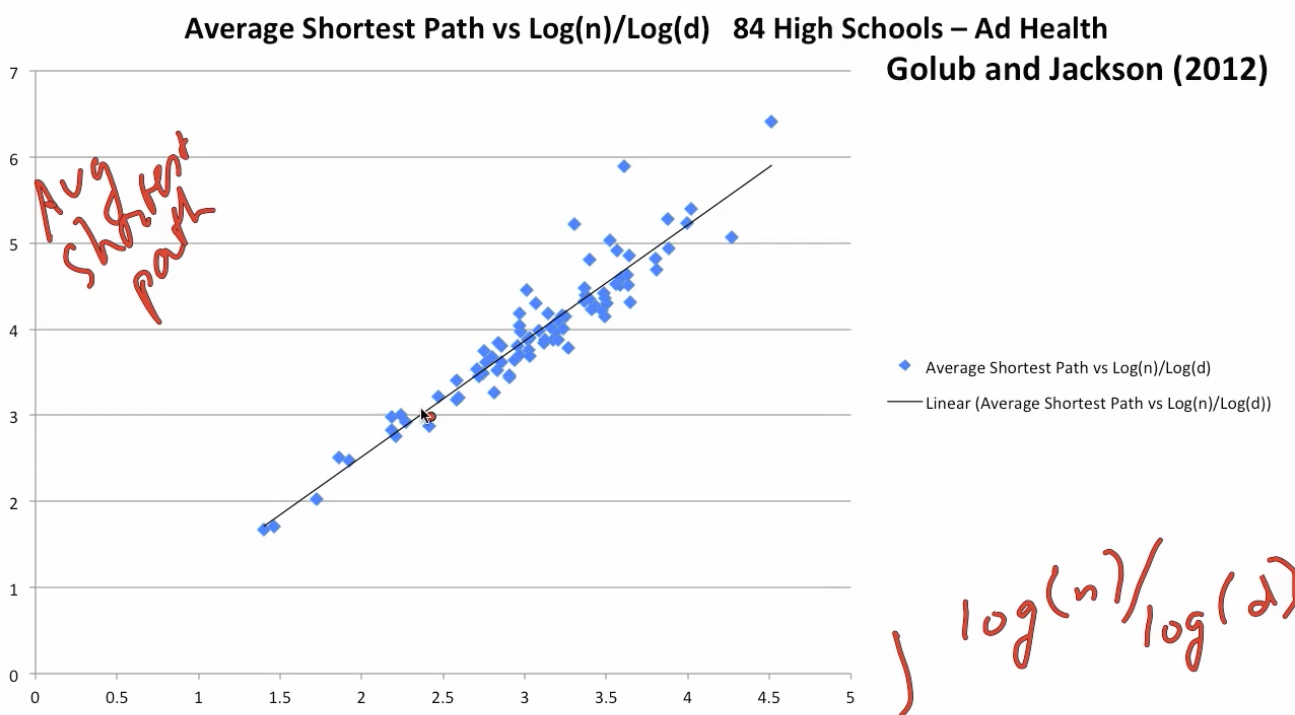# Theorem on Network Structure¶

If $d(n) \ge (1+\epsilon)log(n)$ some $\epsilon \ge 0$ and $\frac{d(n)}{n} \rightarrow 0$:

then for large n, average path length and diameter of this G(n, p) graph are approximately proportional to $\frac{log(n)}{log(d)}$

$$\frac{AvgDist(n)}{log(n) / log(d(n))} \rightarrow ^p 1$$

same for diameter

# Small Worlds/Six Degrees of Seperation¶

• n = 6.7 billion (world population)

• d = 50(friends, relatives...)

• log(n)/log(d) is about 6!

• to get from any individual to any other individual in the world. You actually don't need a lot of hops, you can get there fairly efficiently

# Examine data and diameter¶

• Add Health data set

• Schools vary in average degree and homophily

• Does diameter match log(n)/log(d)?# Erdos Numbers¶

• Number of links in co-authorship network to Erdos

• Had 509 co-authors, more than 1400 papers

• 2004 auction of co-authorship with William Tozier (Erdos #=4) on E-Bay, winner paid > 1000\$

# Density: Average Degree¶

HS Friendships (CJP 09) 6.5

Romances (BMS 03) 0.8

Borrowing (BCDJ 12) 3.2

Co-authors (Newman 01, GLM 06)

• Bio 15.5
• Econ 1.7
• Math 3.9
• Physics 9.3

Facebook (Marlow 09) 120